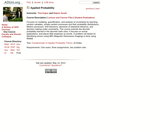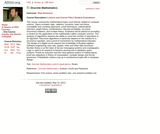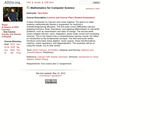# 3 Results

View
Selected filters:
• ArsDigita UniversityConditional Remix & Share Permitted
CC BY-SA
Rating

Focuses on modeling, quantification, and analysis of uncertainty by teaching random variables, simple random processes and their probability distributions, Markov processes, limit theorems, elements of statistical inference, and decision making under uncertainty. This course extends the discrete probability learned in the discrete math class. It focuses on actual applications, and places little emphasis on proofs. A problem set based on identifying tumors using MRI (Magnetic Resonance Imaging) is done using Matlab.

Subject:
Mathematics
Material Type:
Full Course
Homework/Assignment
Lecture
Lecture Notes
Provider:
ArsDigita University
Provider Set:
ArsDigita University
Author:
Rajeev Surati
Tina Kapur
07/05/2018Conditional Remix & Share Permitted
CC BY-SA
Rating

This course covered the mathematical topics most directly related to computer science. Topics included: logic, relations, functions, basic set theory, countability and counting arguments, proof techniques, mathematical induction, graph theory, combinatorics, discrete probability, recursion, recurrence relations, and number theory. Emphasis will be placed on providing a context for the application of the mathematics within computer science. The analysis of algorithms requires the ability to count the number of operations in an algorithm. Recursive algorithms in particular depend on the solution to a recurrence equation, and a proof of correctness by mathematical induction. The design of a digital circuit requires the knowledge of Boolean algebra. Software engineering uses sets, graphs, trees and other data structures. Number theory is at the heart of secure messaging systems and cryptography. Logic is used in AI research in theorem proving and in database query systems. Proofs by induction and the more general notions of mathematical proof are ubiquitous in theory of computation, compiler design and formal grammars. Probabilistic notions crop up in architectural trade-offs in hardware design.

Subject:
Mathematics
Material Type:
Assessment
Full Course
Homework/Assignment
Lecture
Lecture Notes
Syllabus
Provider:
ArsDigita University
Provider Set:
ArsDigita University
Author:
Shai Simonson
07/05/2018Conditional Remix & Share Permitted
CC BY-SA
Rating

A basic introduction to Calculus and Linear Algebra. The goal is to make students mathematically literate in preparation for studying a scientific/engineering discipline. The first week covers differential calculus: graphing functions, limits, derivatives, and applying differentiation to real-world problems, such as maximization and rates of change. The second week covers integral calculus: sums, integration, areas under curves and computing volumes. This is not meant to be a comprehensive calculus course, but rather an introduction to the fundamental concepts. The third and fourth weeks introduce some basic linear algebra: vector spaces, linear transformations, matrices, matrix operations, and diagonalization. The emphasis will be on using the results, not on their proofs.

Subject:
Mathematics
Computer Science
Material Type:
Assessment
Full Course
Homework/Assignment